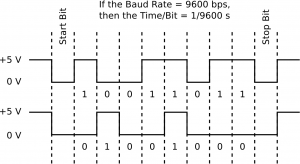by

# Introduction to Arduino Serial Communication

Serial communication is a method for two computers or microcontrollers (or one computer and a microcontroller) to talk to one another. Arduino uses the transistor-transistor logic (TTL) serial protocol. This protocol sends bits using voltages of zero (for a zero bit) and five (for a one bit). Another popular serial protocol is RS-232 which uses +13 V to represent zeros and -13 V to represent ones. In both cases, serial communication involves sending bytes one bit at a time starting with the least significant bit (LSB). Each bit is preceded by a start bit (0 V for TTL serial communication) and immediately followed by a stop bit (5 V for TTL serial communication). Therefore, the figure below shows the signals that would be generated if the bytes 11011001 (top) and 00010010 (bottom) where sent via a serial channel.The figure above incorrectly shows the stop bit as 0V (low). It should be 5V (high).

In order for two devices to communicate via a serial signal, both must be configured with the same baud rate or bits per second (bps) rate. This tells both machines the interval of time between individual bits so they can synchronize. The most common baud rate for Arduino is 9600. In fact, this is the default baud rate when a new serial monitor is opened from the Arduino IDE. Other baud rates are sometimes necessary. For example, communication with GPS modules often uses a baud rate of 4800.

The following sketch demonstrates serial communication between an Arduino Uno and a the serial monitor on a computer. When a character is sent to the Arduino from the serial monitor on a computer, the Arduino reads this byte and immediately will send it back. The `Serial.begin()`, `Serial.available()`, `Serial.read()`, `Serial.print()`, and `Serial.write()` commands are used to start serial communication, test to see if any bytes are available in the serial buffer, read in the next byte in the serial buffer, print a decimal number to the serial monitor, and print the ASCII character corresponding to this decimal number, respectively. The comments in the code should help you figure out what each component does.

``````// Program Name: Serial Echo

// note: serial bytes are read as ASCII
// (American Standard Code for Information Interchange)
// characters

byte myByte; // a variable to store a byte read from the serial
// buffer

void setup() {
Serial.begin(9600); // begin serial communications at 9600 bps
}

void loop() {
if (Serial.available()>0) {
while(Serial.available()>0){ // while bytes remain in the serial
// buffer
// = is ASCII character 61
// 0-9 are ASCII characters 48 to 57
// - is ASCII character 45
// + is ASCII character 43
}
Serial.print("ASCII Character Value of Byte Read: \n");
Serial.write(myByte);
Serial.print('\n');
Serial.print("ASCII Decimal Value of Byte Read: \n");
Serial.print(myByte); // prints ASCII character corresponding
// to myByte
Serial.print('\n');
}
}``````

One important concept to understand is that serial information is sent out of and read into the Arduino one byte at a time. Moreover, each byte that is read into memory corresponds to the decimal ASCII encoding for the ASCII character being received. So, if the character `A` is sent, the value stored in `myByte` would be `65` since this is the decimal code for the ASCII character `A`. For more on ASCII characters and serial communications see this post.

1.1.